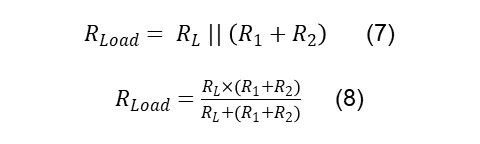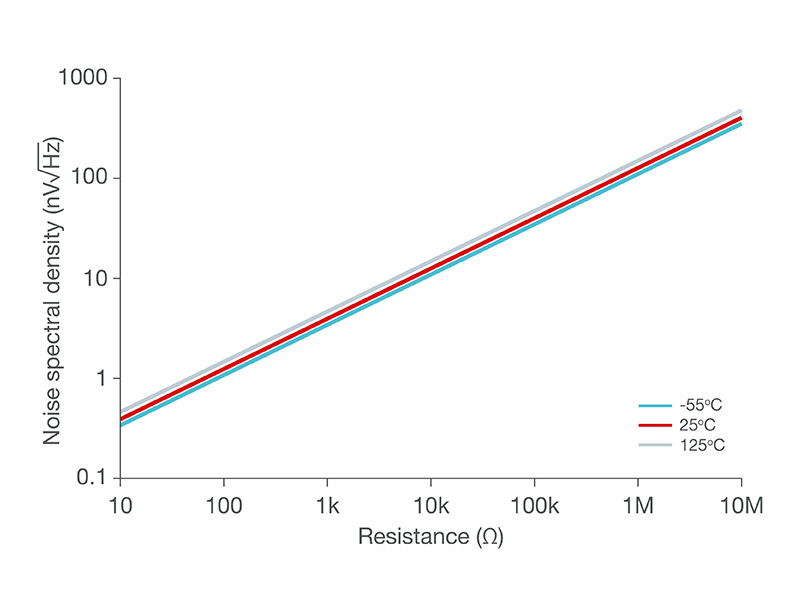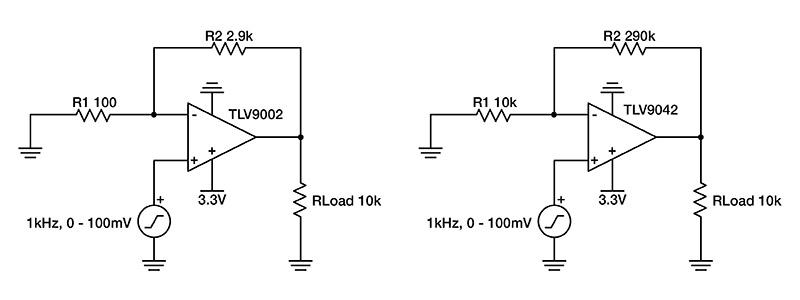# Designing with low-power op amps, part 1: Power-saving techniques for op-amp circuits

Other Parts Discussed in Post: TLV9042, TLV8802, OPA2333, OPA391, TLV9002

In recent years, the popularity of battery-powered electronics has made power consumption an increasing priority for analog circuit designers. With this in mind, this article is the first in a series that will cover the ins and outs of designing systems with low-power operational amplifiers (op amps).

In the first installment, I will discuss power-saving techniques for op-amp circuits, including picking an amplifier with a low quiescent current (IQ) and increasing the load resistance of the feedback network.

### Understanding power consumption in op-amp circuits

Let’s begin by considering an example circuit where power may be a concern: a battery-powered sensor generating an analog, sinusoidal signal of 50 mV amplitude and 50 mV of offset at 1 kHz. The signal needs to be scaled up to a range of 0 V to 3 V for signal conditioning (Figure 1), while saving as much battery power as possible, and that will require a noninverting amplifier configuration with a gain of 30 V/V, as shown in Figure 2. How can you optimize the power consumption of this circuit?Figure 1: Input and output signalsFigure 2: A sensor amplification circuit

Power consumption in an op-amp circuit consists of various factors: quiescent power, op amp output power, and load power. The quiescent power, PQuiescent, is the power needed to keep the amplifier turned on and consists of the op amp’s IQ, which is listed in the product data sheet. The output power, POutput, is the power dissipated in the op amp’s output stage to drive the load. Finally, load power, PLoad, is the power dissipated by the load itself. My colleague, Thomas Kuehl, in his technical article, “Top questions on op-amp power dissipation – part 1,” and a TI Precision Labs video, “Op Amps: Power and Temperature,” define various equations for calculating power consumption for an op amp circuit.

In this example, we have a single-supply op amp with a sinusoidal output signal that has a DC voltage offset. So we will use the following equations to find the total, average power, Ptotal,avg. The supply voltage is represented by V+. Voff is the DC offset of the output signal and Vamp is the output signal’s amplitude. Finally, RLoad is the total load resistance of the op amp. Notice that the average total power is directly related to IQ while inversely related to RLoad.Picking a device with the right IQ

Equations 5 and 6 have several terms and it’s best to consider them one at a time. Selecting an amplifier with a low IQ is the most straightforward strategy to lower the overall power consumption. There are, of course, some trade-offs in this process. For example, devices with a lower IQ typically have lower bandwidth, greater noise and may be more difficult to stabilize. Subsequent installments of this series will address these topics in greater detail.

Because the IQ of op amps can vary by orders of magnitude, it’s worth taking the time to pick the right amplifier. TI offers circuit designers a broad selection range, as you can see in Table 1. For example, the TLV9042, OPA2333, OPA391 and other micropower devices deliver a good balance of power savings and other performance parameters. For applications that require the maximum power efficiency, the TLV8802 and other nanopower devices will be a good fit. You can search for devices with your specific parameters, such as those with ≤10 µA of IQ, using our parametric search.

 Typical specifications TLV9042 OPA2333 OPA391 TLV8802 Supply voltage (VS) 1.2 V-5.5 V 1.8 V-5.5 V 1.7 V-5.5 V 1.7 V-5.5 V Bandwidth (GBW) 350 kHz 350 kHz 1 MHz 6 kHz Typical IQ per channel at 25°C 10 µA 17 µA 22 µA 320 nA Maximum IQ per channel at 25°C 13 µA 25 µA 28 µA 650 nA Typical offset voltage (Vos) at 25°C 600 µV 2 µV 10 µV 550 µV Input voltage noise density at 1 kHz (en) 66 nV/√Hz 55 nV/√Hz 55 nV/√Hz 450 nV/√Hz

Table 1: Notable low-power devices

### Reducing the resistance of the load network

Now consider the rest of the terms in Equations 5 and 6. The Vamp terms cancel out with no effect on Ptotal,avg and Voff is generally predetermined by the application. In other words, you often cannot use Voff to lower power consumption. Similarly, the V+ rail voltage is typically set by the supply voltages available in the circuit. It may appear that the term RLoad is also predetermined by the application. However, this term includes any component that loads the output and not just the load resistor, RL. In the case of the circuit shown in figure 1, RLoad would include RL and the feedback components, R1 and R2. Hence, RLoad would be defined by Equations 7 and 8.By increasing the values of the feedback resistors, you can decrease the output power of the amplifier. This technique is especially effective when Poutput dominates PQuiescent, but has its limits. If the feedback resistors become significantly larger than RL, then RL will dominate RLoad such that the power consumption will cease to shrink. Large feedback resistors can also interact with the input capacitance of the amplifier to destabilize the circuit and generate significant noise.

To minimize the noise contribution of these components, it’s a good idea to compare the thermal noise of the equivalent resistance seen at each of the op-amp’s inputs (see Figure 3) to the amplifier’s voltage noise spectral density. A rule of thumb is to ensure that the amplifier’s input voltage noise density specification is at least three times greater than the voltage noise of the equivalent resistance as viewed from each of the amplifier’s inputs.Figure 3: Resistor thermal noise

### Real-world example

Using these low-power design techniques, let’s return to the original problem: a battery-powered sensor generating an analog signal of 0 to 100 mV at 1 kHz needs a signal amplification of 30 V/V. Figure 4 compares two designs. The design on the left uses a typical 3.3-V supply, resistors not sized with power-savings in mind and the TLV9002 general-purpose op amp. The design on the right uses larger resistor values and the lower-power TLV9042 op amp. Notice that the noise spectral density of the equivalent resistance, approximately 9.667 kΩ, at the TLV9042’s inverting input is more than three times smaller than the broadband noise of the amplifier in order to ensure that the noise of the op amp dominates any noise generated by the resistors.Figure 4: A typical design vs. a power-conscious design

Using the values from Figure 4, the design specifications and the applicable amplifier specifications, Equation 6 can be solved to give Ptotal,avg for the TLV9002 design and the TLV9042 design. For your reading convenience, Equation 6 has been copied here as Equation 9. Equations 10 and 11 show the numeric values of Ptotal,avg for the TLV9002 design and the TLV9042 design, respectively. Equations 12 and 13 show the results.As can be seen from the last two equations, the TLV9002 design consumes more than four times the power of the TLV9042 design. This is a consequence of a higher amplifier IQ, demonstrated in the left terms of Equations 10 and 11, along with smaller feedback resistors, as accounted for in the right terms of Equations 10 and 11. In the case where more IQ and smaller feedback resistors are not needed, implementing the techniques described here can provide significant power savings.

### Conclusion

I’ve covered the basics of designing amplifier circuits for low power consumption, including picking a device with low IQ and increasing the values of the discrete resistors. In part 2 of this series, I’ll take a look at when you can use low-power amplifiers with low voltage supply capabilities.

• Hi ashare,

Thanks for your question!  I would be happy to explain.

When designing a low noise circuit, the circuit designer will usually choose a low-noise op amp.  However, if the resistors used in the circuit are too large, then the noise from the resistors will be greater than the noise from the op amp itself and the benefit of the low-noise amplifier will be lost.

In order to ensure this does not happen, you should choose the equivalent resistance such that the resistor noise density of the resistors is 3x less than the high frequency noise density of the op amp.  The reason for the 3x value is because the total noise of the circuit will be the square root of the sum of squares of all the noise sources.  In other words, if the op amp has 3x the noise density then it will have about 10x the noise contribution because of the sum of squares.

There is a very good presentation on this topic on ti.com.  Here is a link to both the video and the pdf with the slides.  I recommend reviewing “Rule 1” and “Rule 2” at the beginning of the presentation.

Please let me know if you have any further questions!

• Hi Daniel,

Could you elaborate more on your statement with an example - "A rule of thumb is to ensure that the amplifier’s input voltage noise density specification is at least three times greater than the voltage noise of the equivalent resistance as viewed from each of the amplifier’s inputs."?

Thank you.

• Thanks for the feedback John!  You are right in that this example is skewed toward the resistor values saving more power.  In a real-world application, the op amp choice may often be more involved.  You can still go down another order of magnitude and even further with your op amp's quiescent current as compared to the TLV904x devices.  But at that point you really have to consider the bandwidth and noise tradeoffs.  At a first glance, these two amps can be considered for the same application.  So I thought it was a somewhat fair comparison.

Most engineers would probably remember to keep the resistors large for a low-power application.  But, it's nice to have a reminder of just how great the benefits can be...and also that there are a couple factors limiting this approach.

I hope this was a good refresher and that you learned a thing or two along the way!

• Great blog, and I really liked going through the math with an example. I knew that feedback resistors would contribute to power consumption but never sat down to try and calculate their effect. In your example 165µW is save with the lower Iq amp whereas the rest of the power savings, 1.633mW, is saved from the resistor selection.